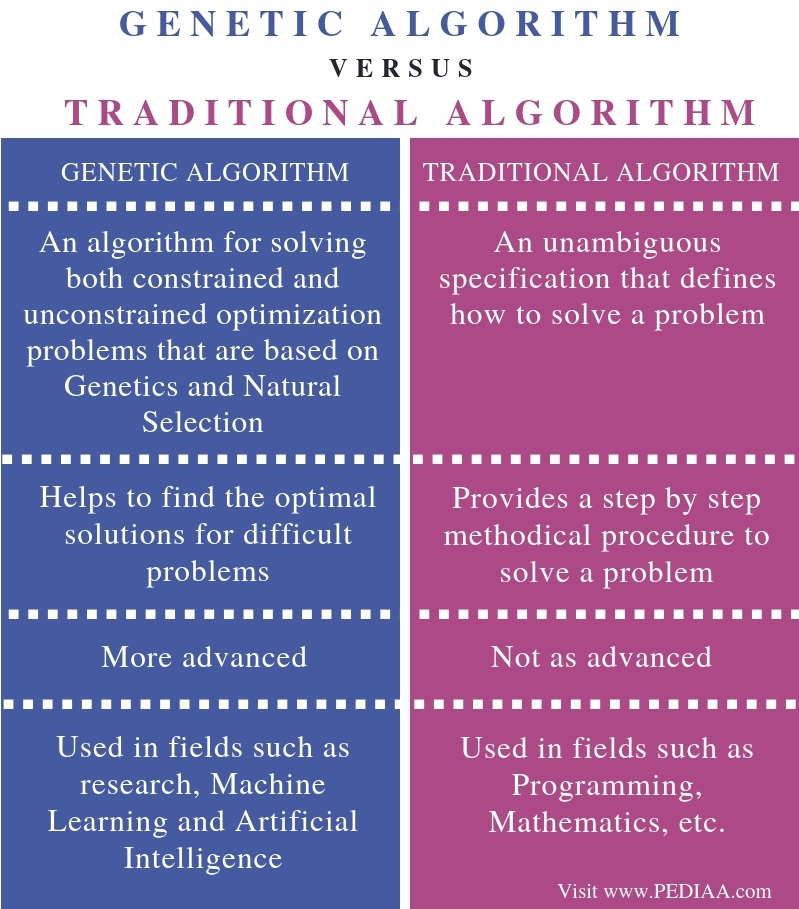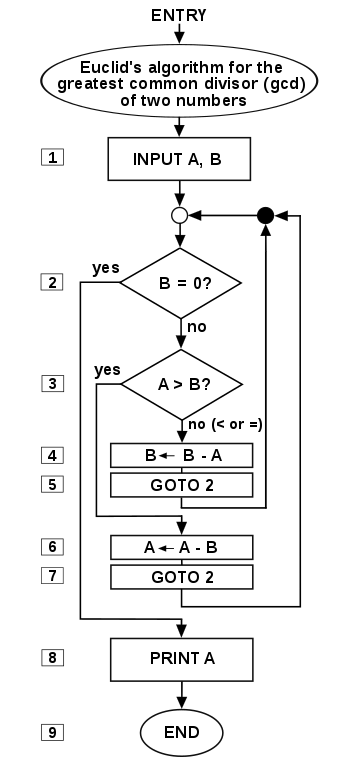# What is the Difference Between Genetic Algorithm and Traditional Algorithm

The main difference between genetic algorithm and traditional algorithm is that the genetic algorithm is a type of algorithm that is based on the principle of genetics and natural selection to solve optimization problems while the traditional algorithm is a step by step procedure to follow in order to solve a given problem.

A procedure is a finite sequence of instructions, where each can be carried out in a finite amount of time. An algorithm is a step by step procedure to solve a problem. There is an algorithm to solve every task. Algorithms are especially helpful when solving complex problems. General algorithms are also known as traditional algorithms. Genetic algorithm is a special type of algorithm which is mainly used in areas such as Artificial intelligence.

### Key Areas Covered

1. What is Genetic Algorithm
– Definition, Functionality
– Definition, Functionality
3. What is the Difference Between Genetic Algorithm and Traditional Algorithm
– Comparison of Key Differences

### Key Terms

Algorithm, Genetic Algorithm, Machine Learning, Search, Sort, Divide and Conquer, Traditional Algorithm## What is Genetic Algorithm

Genetic algorithm refers to a type of algorithms that are based on Genetics and Natural Selection. It is similar to the process of the species which can adapt to changes that occur in the environment and are capable of surviving.  In other words, it is based on biological evolution.

Furthermore, this algorithm continuously modifies the population of individual solutions. At each and every step, it chooses individuals randomly from the current population as parents and creates children for the next generation. Going from generation to generation helps in finding the optimal solution.

Generic Algorithm mainly uses three types of rules at each step to create the next generation using the current population.

Selection rules – It chooses individuals (parents) that contribute to the population of the next generation.

Crossover rules – It joins two parents to generate a child for the next generation.

Mutation rules – It applies changes to individual parents to create children.

Genetic algorithm helps to find the optimal solution or near-optimal solution to a problem. It avoids the usual time required to solve a particular problem. Moreover, it is mainly used in fields such as research and machine learning to solve optimization problems.

Traditional Algorithms refers to general algorithms we use to solve problems. It is a methodical procedure to solve a given problem. There can be several algorithms to solve a problem. Therefore, it is important to analyze each and find the required time, space etc. to select the best algorithm to solve the problem.

A traditional algorithm must be clear. It should have 0 or more number of well-defined inputs and 1 or more well-defined outputs. It should also be capable of finding solutions with available resources. Furthermore, it should complete the task after some finite number of steps.Following are some common traditional algorithms.

Search – These algorithms help to search a particular item in a data structure. (Linear search, Binary search)

Sort – These algorithms help to sort a set of elements in a specific order. (Bubble sort, Selection sort, Insertion sort)

Divide and Conquer – It refers to dividing a large problem into small subproblems and solving the subproblems in order to find the solution to the original problem.

## Difference Between Genetic Algorithm and Traditional Algorithm

### Definition

Genetic algorithm is an algorithm for solving both constrained and unconstrained optimization problems that are based on Genetics and Natural Selection while traditional algorithm is an unambiguous specification that defines how to solve a problem. Thus, this is the main difference between genetic algorithm and traditional algorithm.

### Usage

The specific use of each algorithm is an important difference between genetic algorithm and traditional algorithm. That is; the genetic algorithm helps to find the optimal solutions for difficult problems while traditional algorithm provides a step by step methodical procedure to solve a problem.

### Complexity

Another difference between genetic algorithm and traditional algorithm is that a genetic algorithm is more advanced than a traditional algorithm.

### Applications

Genetic Algorithm is used in fields such as research, Machine Learning and, Artificial Intelligence. Traditional algorithm is used in fields such as Programming, Mathematics, etc. Hence, this is also an important difference between genetic algorithm and traditional algorithm.

### Conclusion

In addition to traditional algorithms, there is a special type of algorithm called genetic algorithms. The main difference between genetic algorithm and traditional algorithm is that genetic algorithm is a type of algorithm that is based on the principle of genetics and natural selection to solve optimization problems while traditional algorithm is a step by step procedure to follow, in order to solve a given problem. Searching, sorting algorithms etc. are traditional algorithms while genetic algorithms are more complex and used in areas such as artificial intelligence and research.

##### Reference:

1. “Select a Web Site.” What Is the Genetic Algorithm? – MATLAB & Simulink, Available here.
2. “Algorithm.” Wikipedia, Wikimedia Foundation, 18 Mar. 2019, Available here.

##### Image Courtesy:

1. “Euclid flowchart” By Somepics – Own work (CC BY-SA 4.0) via Commons Wikimedia